# 一种基于模板匹配的线性特征混合像元的亚像元定位的新方法New Template Matching Method for Subpixel Mapping of Linear Feature Mixed Pixels

DOI: 10.12677/JISP.2019.83024, PDF, HTML, XML, 下载: 473  浏览: 888  国家自然科学基金支持

Abstract: By analyzing the problems of subpixel mapping algorithm based on template matching, a linear feature sub-pixel mapping algorithm based on improved template matching is proposed. Based on the correlation coefficient selection template, two new template selection methods are proposed: 1) Further determining the template by fitting a line with linear feature mixed pixels eight neigh-borhood, to some extent, reduces the scope of template selection; 2) In order to avoid the uncer-tainty of template selection, the best template is obtained through line fitting on the mixed pixels eight neighborhoods and the template and calculating the linear correlation. Furthermore, com-bined with pixel gravity, the sub-pixel mapping problem of linear feature pixel containing three or more ground objects is solved. The experiment results show that the proposed method can improve the sub-pixel mapping accuracy.

1. 引言

2. 含线特征混合像元亚像元定位的模板匹配法

2.1. 含线特征混合像元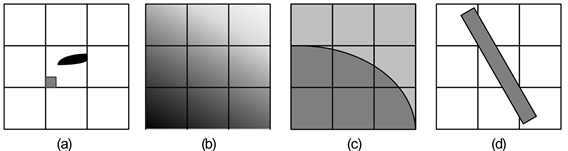Figure 1. Four different types of mixed pixels. (a) Sub-pixel target; (b) Dense mixed pixels; (c) Boundary mixed pixel; (d) Linear target

2.2. 亚像元定位的模板匹配法

1) 对每个混合像元，确定像元尺度下最佳匹配的线状模板。Figure 2. 3 × 3 template

${T}_{k}=\left[\begin{array}{ccc}t\left(-1,-1\right)& t\left(-1,0\right)& t\left(-1,1\right)\\ t\left(0,-1\right)& t\left(0,0\right)& t\left(0,1\right)\\ t\left(1,-1\right)& t\left(1,0\right)& t\left(1,1\right)\end{array}\right]$ (1)

${r}_{i,j,k}=\frac{{\sum }_{m=-w}^{w}{\sum }_{n=-w}^{w}\text{ }{T}_{k}\left(m,n\right)\cdot {S}_{c}\left(i+m,j+n\right)}{\sqrt{{\sum }_{m=-w}^{w}{\sum }_{n=-w}^{w}\text{ }\text{ }{S}_{c}{\left(i+m,j+n\right)}^{2}\cdot {\sum }_{m=-w}^{w}{\sum }_{n=-w}^{w}\text{ }{T}_{k}{\left(m,n\right)}^{2}}}$ (2)

2) 利用最优模板 ${T}_{k}$ 对混合像元Pij中第c类地物进行定位。

3) 像元内其他亚像元类别确定

2.3. 存在的问题

1) 直接根据相关系数ri,j,k进行模板选择时最大ri,j,k对应的模板可能不唯一，如何从多个匹配的模板中选出唯一的最优模板仍是个问题；

2) 当混合像元内包含三种及以上地物时，用随机方法确定像元内其他亚像元类别不够准确。

3. 两种最佳匹配模板确定的新方法

3.1. 基于拟合直线的最佳匹配模板确定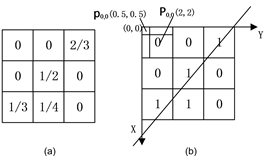Figure 3. Schematic diagram of line fitting. (a) Eight neighborhood abundance map of mixed pixels of class c; (b) Linear fitting after binarization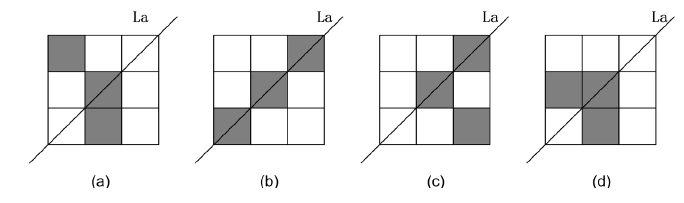Figure 4. 4 results of line fitting

$d\left({P}_{mn},{L}_{a}\right)=|\frac{A{x}_{mn}+B{y}_{mn}+C}{\sqrt{{A}^{2}+{B}^{2}}}|$ (3)

NT值最大的模板为最优模板。图4给出了图2中部分模板的直线拟合结果，其中各模板中直线所经过的灰色区域个数NT分别为1，3，2，1，图4(b)所示模板为最优模板。

3.2. 标准相关匹配结合直线拟合相关性选择最佳模板

SC-PLF方法虽然进一步缩小了模板选择的范围，但是对于模板唯一性仍无法保证。为此本节提出了标准相关匹配结合直线拟合相关性(Line Fitting Correlation after Standard Correlation, SC-LFC)的方法，在基于标准相关性的模板匹配选择方法选择出的若干模板基础上，分别对所选的模板和含线特征混合像元邻域进行直线拟合，并计算各模板和像元拟合直线间的相关性，可以保证模板选择的唯一性，算法主要思想是：

1) 对混合像元Pi,j及其邻域像元根据地物 的丰度进行二值化，拟合直线 ${L}_{a}=k1\cdot x+b1$

2) 对经过SC算法选择出的各模板，根据 $t\left(m,n\right)=1$ 的位置拟合直线 ${L}_{i}=k2\cdot x+b2,i=1,2,\cdots ,k$

3) 利用式(4)计算La和直线 ${L}_{1},{L}_{2},\cdots ,{L}_{k}$ 的相关性：

$L{C}_{i}=w1\left(k1-ki\right)+w2\left(b1-bi\right),\text{\hspace{0.17em}}\text{\hspace{0.17em}}i=1,2,\cdots ,k$ (4)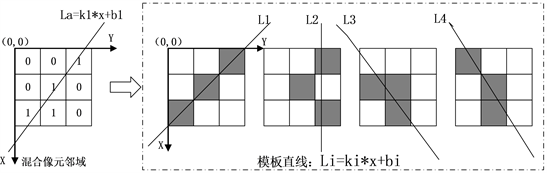Figure 5. Result of line fitting

4. 改进的含线特征混合像元亚像元定位流程

$N\left({P}_{ab}\right)=\left\{{P}_{ij}|\left(\frac{a}{s}\ne i\vee \frac{b}{s}\ne j\right)\wedge \left(|\frac{a}{s}-i|\le 1\wedge |\frac{b}{s}-j|\le 1\right)\right\}$ (5)

${P}_{ab}\left(c\right)=\text{avg}\left\{\frac{{S}_{c}\left(x,y\right)}{d\left({P}_{ij},{P}_{ab}\right)}|{P}_{ij}\in N\left({P}_{ab}\right)\right\}$ (6)

$d\left({P}_{ab},{P}_{ij}\right)=\sqrt{{\left[a+0.5-s×\left(i+0.5\right)\right]}^{2}+{\left[b+0.5-s×\left(j+0.5\right)\right]}^{2}}$ (7)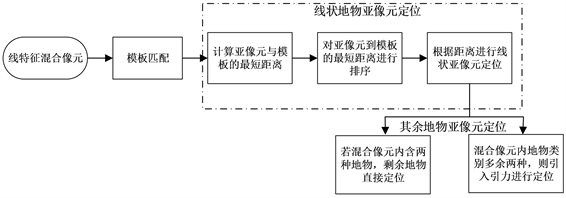Figure 6. Schematic diagram of the subpixels mapping process of linear feature mixed pixels

5. 实验与分析Table 1. Description of the subpixel mapping algorithm of linear feature mixed pixels

5.1. 实验数据

5.1.1. 仿真数据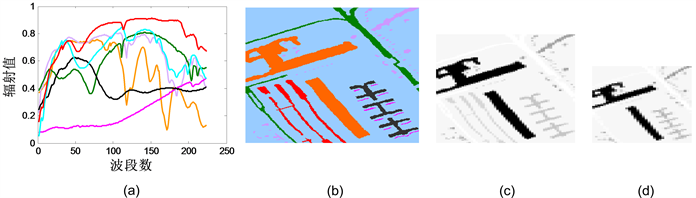Figure 7. Synthetic dataset. (a) 7 kinds of ground spectrum; (b) Ground truth; (c) 90 × 60 synthetic map; (d) 54 × 36 synthetic map

5.1.2. 真实数据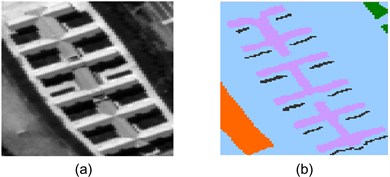Figure 8. Real hyperspectral dataset. (a) Grayscale map of the 37th band; (b) Ground truth

5.2. 混合像元内线状地物亚像元定位实验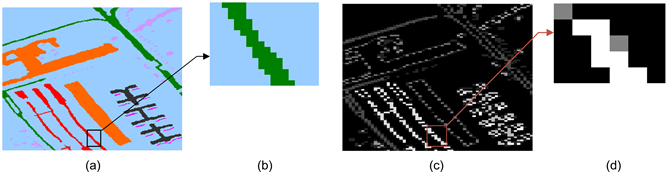Figure 9. Experimental data. (a) Schematic diagram of subgraph capture; (b) Scaled subgraph; (c) Line feature detection result of graph (a); (d) Detection result of graph (b)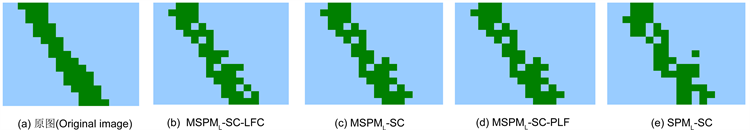Figure 10. Result of line feature mixed pixels mapping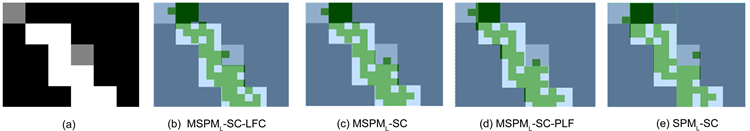Figure 11. Result of line detection (a) and result of line future mixed pixels mapping (b) - (e)Table 2. Comparison of mixed pixels mapping results of each line feature algorithm (without optimization)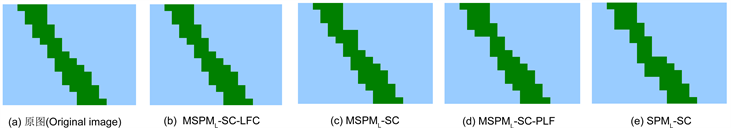Figure 12. Result of subpixel mapping of Figure 9(b)Table 3. Subpixel mapping result of each algorithm

5.3. 亚像元定位实验

5.3.1. 仿真数据实验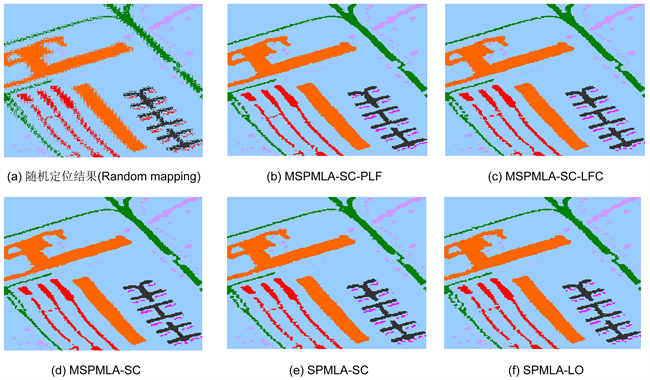Figure 13. Subpixel mapping result of reconstruction scale of 3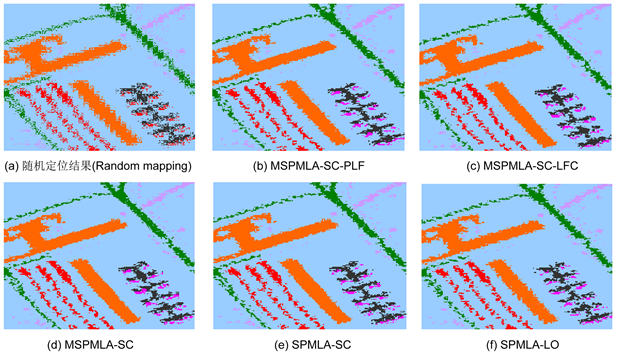Figure 14. Subpixel mapping result with reconstruction scale of 5Table 4. Experiment result with reconstruction scale of 3Table 5. Experiment result with reconstruction scale of 5

5.3.2. 真实数据实验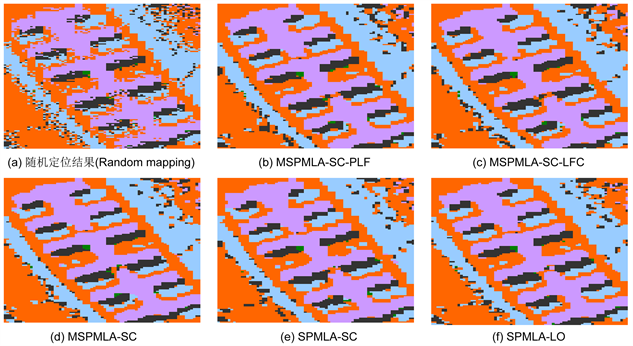Figure 15. Result of subpixel mappingTable 6. Experiment result with reconstruction scale of 3

6. 结论

NOTES

*通讯作者。

  Tong, X., Xu, X., Plaza, A., et al. (2016) A New Genetic Method for Subpixel Mapping Using Hyperspectral Images. IEEE Journal of Selected Topics in Applied Earth Observations and Remote Sensing, 9, 4480-4491. https://doi.org/10.1109/JSTARS.2015.2496660  Bioucas-Dias, J.M., Plaza, A., et al. (2012) Hyperspectral Unmixing Overview: Geometrical, Statistical, and Sparse Regression-Based Approaches. IEEE Journal of Selected Topics in Applied Earth Observations and Remote Sensing, 2, 354-379.https://doi.org/10.1109/JSTARS.2012.2194696  陈晋, 马磊, 陈学泓. 混合像元分解技术及其进展[J]. 遥感学报, 2016, 20(5): 1102-1109.  赵春晖, 成宝芝, 杨伟超. 利用约束非负矩阵分解的高光谱解混算法[J]. 哈尔滨工程大学学报, 2012, 33(3): 377-382.  Athinkson, P.M. (1997) Mapping Sub-Pixel Boundaries from Remotely Sensed Images. Innovations in GIS, 4, 166-180.  Xu, X., Zhong, Y. and Zhang, L. (2014) A Sub-Pixel Mapping Method Based on an Attraction Model for Multiple Shifted Remotely Sensed Images. Neurocomputing, 134, 79-91. https://doi.org/10.1016/j.neucom.2012.12.078  Wu, S., Chen, Z., Ren, J., et al. (2018) An Improved Subpixel Mapping Algorithm Based on a Combination of the Spatial Attraction and Pixel Swapping Models for Multispectral Remote Sensing Imagery. IEEE Geoscience and Remote Sensing Letters, 15, 1-5. https://doi.org/10.1109/LGRS.2018.2825472  许雄, 钟燕飞, 张良培, 等. 基于空间自相关BP神经网络的遥感影像亚像元定位[J]. 测绘学报, 2011, 40(3): 307-311.  吴柯, 牛瑞卿, 沈焕峰, 等. 结合超分辨率重建的神经网络亚像元定位方法[J]. 中国图象图形学报, 2010, 15(11): 1681-1687.  王群明. 基于软硬属性转换的遥感图像亚像元定位算法[J]. 测绘学报, 2016, 45(4): 503-503.  Wang, P., Wang, L. and Chanussot, J. (2016) Soft-then-Hard Subpixel Land Cover Mapping Based on Spatial-Spectral Interpolation. IEEE Geoscience and Remote Sensing Letters, 13, 1851:1854. https://doi.org/10.1109/LGRS.2016.2614810  赵辽英, 范明阳, 厉小润, 等. 基于目标优化的高光谱图像亚像元定位[J]. 中国图象图形学报, 2016, 21(6): 823-833.  Chen, S., Li, X. and Zhao, L. (2017) Subpixel Mapping Method of Hyperspec-tral Images Based on Modified Binary Quantum Particle Swarm Optimization. Journal of Electrical and Computer Engineering, No. 3, 1-17. https://doi.org/10.1155/2017/2683248  Zhong, Y. and Zhang, L. (2013) Sub-Pixel Mapping Based on Artificial Immune Systems for Remote Sensing Imagery. Pattern Recognition, 46, 2902-2926. https://doi.org/10.1016/j.patcog.2013.04.009  Xu, X., Zhong, Y. and Zhang, L. (2013) Adaptive Subpixel Mapping Based on a Multiagent System for Remote-Sensing Imagery. IEEE Transactions on Geoscience and Remote Sensing, 52, 787-804. https://doi.org/10.1109/TGRS.2013.2244095  Ge, Y., Chen, Y., Stein, A., et al. (2016) Enhanced Subpixel Mapping with Spatial Distribution Patterns of Geographical Objects. IEEE Transactions on Geoscience and Remote Sensing, 54, 2356-2370. https://doi.org/10.1109/TGRS.2015.2499790  Fisher, P. (1997) The Pixel: A Snare and a Delusion. International Journal of Remote Sensing, 18, 679-685. https://doi.org/10.1080/014311697219015  Chang, C.I. (2017) Adaptive Linear Spectral Mixture Analysis. IEEE Transac-tions on Geoscience and Remote Sensing, 55, 1240-1253. https://doi.org/10.1109/TGRS.2016.2620494  Heinz, D.C. and Chang, C.I. (2002) Fully Constrained Least Squares Linear Spectral Mixture Analysis Method for Material Quantification in Hyper-spectral Imagery. IEEE Transactions on Geoscience and Remote Sensing, 39, 529-545. https://doi.org/10.1109/36.911111  王丽姣, 厉小润, 赵辽英. 快速实现基于单形体体积生长的端元提取算法[J]. 光学学报, 2014, 34(11): 308-314.  Erturk, A., Gullu, M.K., Cesmeci, D., et al. (2014) Spatial Resolution Enhancement of Hyper-spectral Images Using Unmixing and Binary Particle Swarm Optimization. IEEE Geoscience and Remote Sensing Letter, 11, 2100-2104. https://doi.org/10.1109/LGRS.2014.2320135  Ling, F. and Fu, B. (2009) Super-Resolution Mapping of Urban Buildings with Remotely Sensed Imagery Based on Prior Shape Information. Joint Urban Remote Sensing Event, Shanghai, 20-22 May 2009, 1-5. https://doi.org/10.1109/URS.2009.5137529  Rwanga, S.S. and Ndambuki, J.M. (2017) Accuracy Assessment of Land Use/Land Cover Classification Using Remote Sensing and GIS. International Journal of Geosciences, 8, 611-622. https://doi.org/10.4236/ijg.2017.84033